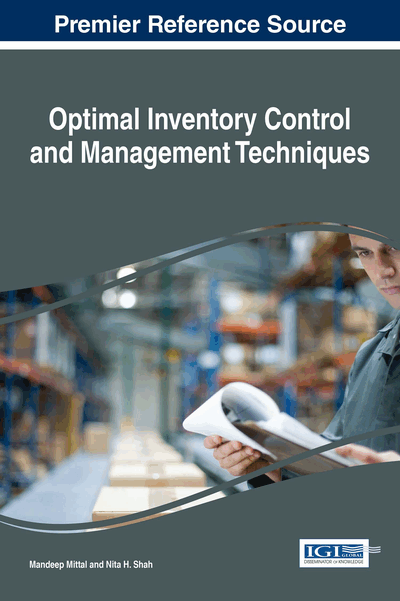# EPQ Inventory Models under mλ-Measure

Hardik N. Soni (Chimanbhai Patel Post Graduate Institute of Computer Applications, India) and Shivangi Suthar (Survey of India, India)
DOI: 10.4018/978-1-4666-9888-8.ch008

## Abstract

This chapter considers an EPQ model with and without shortages under linear combination of Possibility measure and Necessity measure. Based on the possibility measure and necessity measure, m? -measure is introduced and some important properties are discussed. To capture the real life situation, various EPQ model parameters for instance, demand, setup cost, holding cost and backorder cost are characterized as Trapezoidal Fuzzy Number. Two fuzzy chance-constrained programming models are constructed under m?-measure. The objective is to determine optimistic and pessimistic values of the fuzzy objective function with some predefined degree of m?-measure. Using fuzzy arithmetical operations under Function Principle, the fuzzy problem is first transferred to an equivalent crisp problem. An analytical approach is developed to resolve the reduced models. To investigate the characteristics of the proposed model and to obtain the optimal decision under different situations, numerical illustrations are presented along with a sensitivity analysis.
Chapter Preview
Top

## Introduction

The economic production quantity (EPQ) model has been widely accepted and implemented by academicians as well as the business organizations. The EPQ model can be applied to a problem where a product is manufactured on a single facility in order to meet deterministic demand over an infinite planning horizon. The classical EPQ models are mainly developed in deterministic environment, wherein the demand and cost parameters are considered as constant. However, due to floating nature of marketplaces, these assumptions are not realistic. In a real production inventory system, the demand rate and cost parameters are not always fixed, and they often have slight deviation from one cycle to another. Typically, stochastic techniques and statistics methods have been employed to model the uncertainty of inventory parameters. However, statistical inferences are not reliable and not enough to reflect the fluctuation of the inventory related parameters. In most cases, the gauge of demand and cost parameters is often based on the experiences and subjective judgments of the inventory planner, and presented as linguistic expressions, such as “demand belongs to the interval with membership degree” and “holding cost is likely between 15% and 30%”. Thus, to quantify non-stochastic uncertainty in inventory system, it is better to make use of possibility theory rather than probability theory.

There is a vast literature on production inventory problems under fuzzy framework with various settings. The worth mentioning articles in this context are Chang (1999), Chen and Hsieh (1999), Hsieh (2002), De et al. (2003), Chen et al. (2005, 2007), Lee and Chiang (2007), Chen and Chang (2008), Bera et al. (2009), Soni and Shah (2011), Jana et al. (2014) and so forth. Some researchers (e.g. Maity & Maiti (2007), Wang and Teng (2009), Bag and Chakraborty (2014), and so on) employed possibility and/or necessity as the basic measure of the occurrence of the fuzzy event and established optimal policies for inventory system by framing fuzzy Chance Constraint Programming (CCP). In decision making process under fuzzy environment, generally, an optimistic decision maker (DM) chooses possibility to measure a chance of fuzzy event otherwise he selects necessity measure. However, it is necessary to point out that a fuzzy event may fail even though its possibility achieves 1, and hold even though its necessity is 0 (cf. Gao and Liu (2001)). Consequently, high level of confidence in possibility measure does not guarantee the occurrence of fuzzy event. Moreover, if the decision-maker to be eclectic, then a need arises to make use of a combination of both possibility and necessity measure. In this regard, Yang and Imamura (2008) proposed mλ-measure which is a linear combination of possibility measure and necessity measure. Pursuing the notion of mλ-measure, Soni and Joshi (2015) formulated fuzzy EOQ model with backorder. They constructed fuzzy CCP model to find critical values for fuzzy total cost along with optimal ordering policies.

This work is aim to propose an EPQ model with and without backorder in the fuzzy sense, wherein demand, setup cost, holding cost and backorder cost are characterized as Trapezoidal Fuzzy Number (TrFN). Making use of mλ-measure, two fuzzy CCP models are constructed for each EPQ model to determine optimistic and pessimistic returns (cost). An objective function is optimized with some predefined degree of mλ-measure and accordingly the problem is transferred to an equivalent crisp problem and an analytical approach is developed to resolve the reduced models. All the approaches are illustrated with some numerical data in conjunction with sensitivity analysis.

## Complete Chapter List

Search this Book:
Reset